Next: Stochastic sampling Up: Methods for approximating the Previous: Methods for approximating the

Subsections

### Point estimates

The most efficient and least accurate approximation is, in general, a point estimate of the posterior probability. It means that only the model with highest probability or probability density is used for making the predictions and decisions. Whether the accuracy is good depends on how large a part of the probability mass is occupied by models which are similar to the most probable model.

The two point estimates in wide use are the maximum likelihood (ML) and the maximum a posteriori (MAP) estimator. The ML estimator neglects the prior probability of the models and maximises only the probability which the model gives for the observation. The MAP estimator chooses the model which has the highest posterior probability mass or density.

One should be particularly careful when using the MAP estimator with probability densities. The MAP estimator is useful in cases where the second order curvature of the logarithm of the posterior probability density with respect to the model parameters is roughly constant for all models. Then the widths of the peaks of the posterior density are roughly equal and probability mass around any of the models is proportional to the probability density of the model. The second order curvature can be affected by the parameterisation of the model, but in general it depends also on the observations.

For the real valued latent variable models considered in this thesis, the MAP estimators cannot be used as such. The models include products of unknown quantities, weights and factors in this case, which means that by increasing the value of one variable, the value of another variable can be decreased. This scaling does not change the model but the density of the first variable decreases and the second value increases. For each observation, a new set of values is estimated for factors which means that typically the number of unknown values for factors is far greater than the number of unknown values for weights. If the MAP estimates were used for factor analysis models, the result would be that the weights of the model would grow and the values of the factors shrink. The resulting low density of the weights would be overwhelmed by the high density of the factors. In other words, MAP estimation would find the values of the weights which give the highest density for the factors, but would not say much about the posterior probability mass of the model because the high density would be obtained at the cost of narrow posterior peaks of the factors.

In any case, the use of a point estimate will cause a phenomenon called overfitting. Most people are familiar with the concept at least in the context of fitting polynomials to observations. Using only the best model means being excessively confident that the best fit is the correct one. In the case of probability densities, for instance, all probability mass is in models which have a poorer fit than the best model. This means that the optimal prediction based on the full posterior density will necessarily be less confident about the fit than a prediction based on only the best'' model.

#### EM algorithm.

The expectation-maximisation (EM) algorithm  is often used for learning latent variable models, including the factor analysis model . It is a mixture of point estimation and analytic integration over posterior density. The EM algorithm is useful for latent variable models if the posterior probability of the latent variables can be computed when other parameters of the model are assumed to be known.

The EM algorithm was developed for maximum likelihood parameter estimation from incomplete data. Let us denote the measured data by x, the missing data by y and the parameters by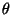. The algorithm starts with an estimate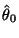and alternates between two steps, called E-step for expectation and M-step for maximisation. In the former, the conditional probability distribution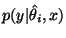of the missing data is computed given the current estimate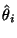of the parameters and in the latter, a new estimate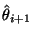of the parameters is computed by maximising the expectation of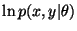over the distribution computed in the E-step.

It can be proven that this iteration either increases the probability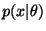or leaves it unchanged. The usefulness of the method is due to the fact that it is often easier to integrate the logarithmic probabilitythan probability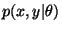which would be required ifwere maximised directly.

The EM algorithm applies to latent variable models when the latent variables are assumed to be the missing data. When compared to simple point estimation, the benefit of the method is that fewer unknown variables are assigned a point estimate, thus alleviating the problems related to overfitting.Next: Stochastic sampling Up: Methods for approximating the Previous: Methods for approximating the
Harri Valpola
2000-10-31